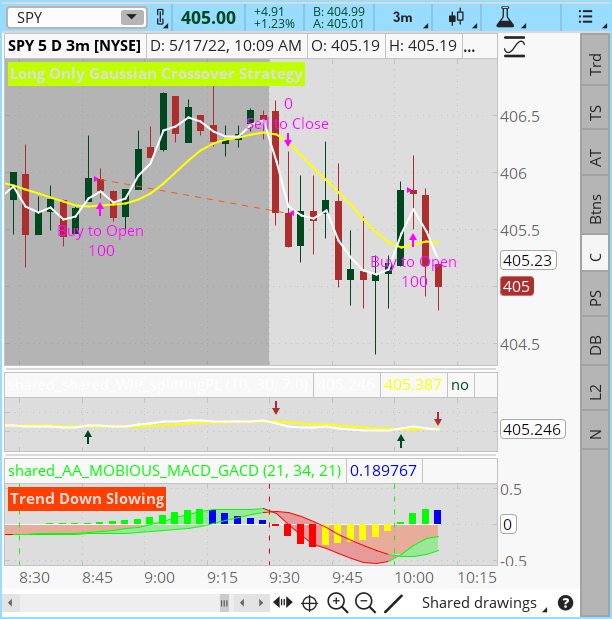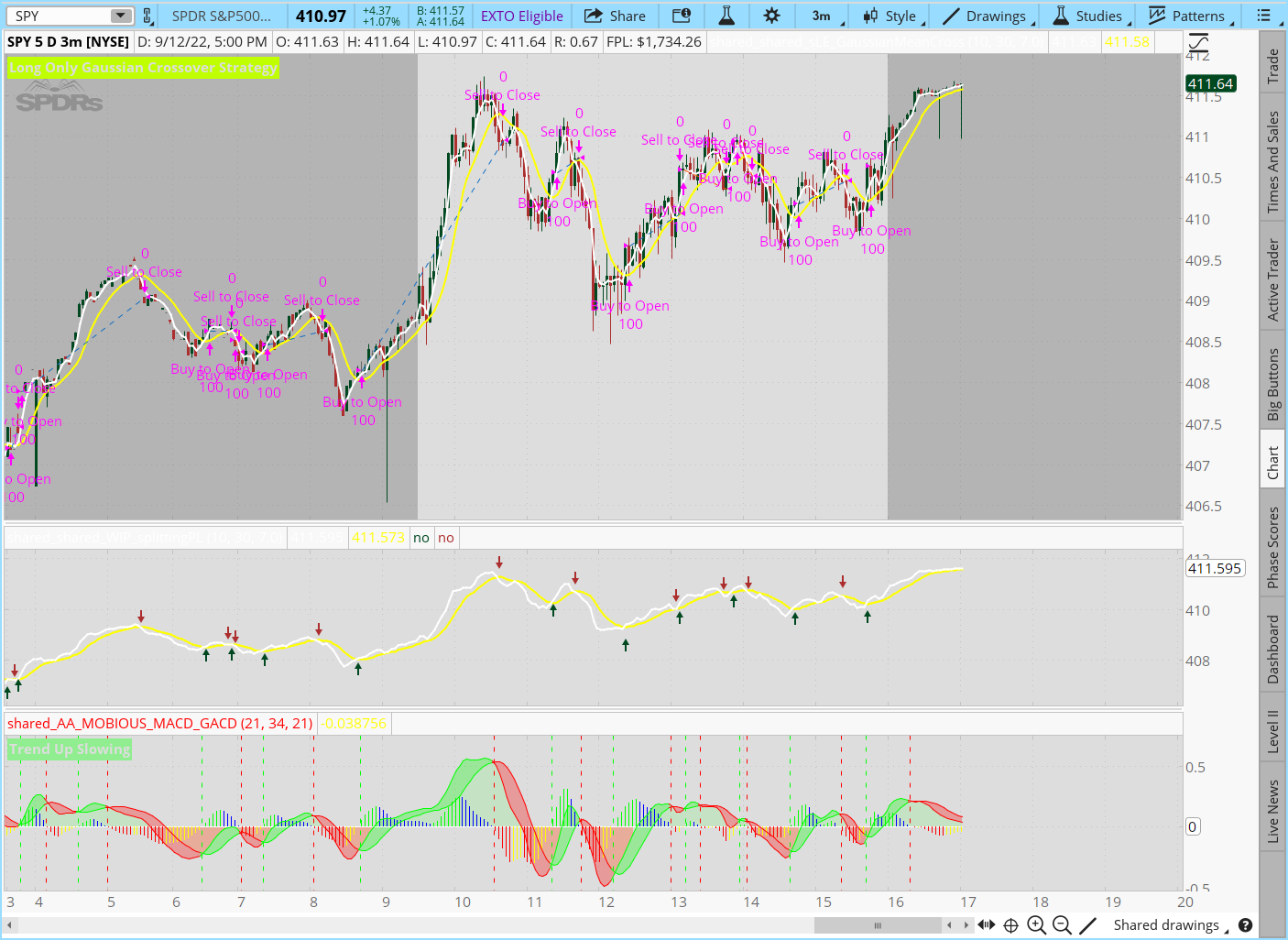# Upper Gaussian Mean crossovers with lower macd/gacd

#### J007RMC

##### Well-known member
2019 Donor
https://tos.mx/c4omXqhOriginal study includes pl
https://tos.mx/OWGLln5

Last edited by a moderator:
•The first of the lower two indicators above is a gaussian mean cross with arrows and so I paired it with the WT_LB Short Name TV and I'm getting good signals both short and long where I take confirmation if present from the WT_LB Short Name TV.. today I ran a 1min and a 512t I like what I'm seeing with the indicator minus the labels.

Code:
``````  # GaussianMeanCrossWithProfitability_JQ

declare lower;
#plot Data = close;
input GaussianLength = 5;
input GaussianLengthLong = 20;
input betaDev = 7.0; #hint betaDev: adjust this value till the label indicates a minimum of 68.2% of closes

# Trying to achieve Normal Distribution
# Mobius
# V01.09.2015
def c;
def w;
def beta;
def alpha;
def G;
plot GaussianMean;
GaussianMean.SetDefaultColor(Color.WHITE);
GaussianMean.SetLineWeight(2);
c = close;
w = (2 * Double.Pi / GaussianLength);
beta = (1 - Cos(w)) / (Power(1.414, 2.0 / betaDev) - 1 );
alpha = (-beta + Sqrt(beta * beta + 2 * beta));
G = Power(alpha, 4) * c +
4 * (1 – alpha) * G – 6 * Power( 1 - alpha, 2 ) * G +
4 * Power( 1 - alpha, 3 ) * G - Power( 1 - alpha, 4 ) * G;
GaussianMean = G;

#*******************************************************************************;
# Trying to achieve Normal Distribution
# Mobius
# V01.09.2015
def c2;
def w2;
def beta2;
def alpha2;
def G2;
plot GaussianMeanLong;
GaussianMeanLong.SetDefaultColor(Color.YELLOW);
GaussianMeanLong.SetLineWeight(2);
c2 = close;
w2 = (2 * Double.Pi / GaussianLengthLong);
beta2 = (1 - Cos(w2)) / (Power(1.414, 2.0 / betaDev) - 1 );
alpha2 = (-beta2 + Sqrt(beta2 * beta2 + 2 * beta2));
G2 = Power(alpha2, 4) * c2 +
4 * (1 – alpha2) * G2 – 6 * Power( 1 - alpha2, 2 ) * G2 +
4 * Power( 1 - alpha2, 3 ) * G2 - Power( 1 - alpha2, 4 ) * G2;
GaussianMeanLong = G2;
## End Gaussian Codes
#    ---------------------------------------------------
## Begin Plots
plot BullishCross = GaussianMean crosses above GaussianMeanLong;
BullishCross.SetPaintingStrategy(PaintingStrategy.BOOLEAN_ARROW_UP);
BullishCross.SetDefaultColor(Color.UPTICK);
plot BearishCross = GaussianMean crosses below GaussianMeanLong;
BearishCross.SetPaintingStrategy(PaintingStrategy.BOOLEAN_ARROW_DOWN);
BearishCross.SetDefaultColor(Color.DOWNTICK);

# Total Cross Count in Bubbles
# TsL for fredw
# Nube 9.24.18
# universals
def bn = BarNumber();
#def c = close;
def na = Double.NaN;
# variables
def upCross = GaussianMean crosses above GaussianMeanLong;
def upCrossCt = CompoundValue(1,
if upCross
then upCrossCt + 1
else upCrossCt, 0);
def currentUpCrossCount = HighestAll(if !IsNaN(c) && IsNaN(c[-1])
then upCrossCt else na);
def upCrossBar = if upCross
then bn else upCrossBar;
# plots
# alternative version for those who prefer to also use GetValue()
#def currentBar = HighestAll(if !IsNaN(c) && IsNaN(c[-1])
# then bn else na);
#def getUpCount = GetValue(upCrossCt, bn - currentBar);

## ----- ----- ----- ----- ----- ----- ----- ----- ----- -----
## ProfitLossSnippet_v04_JQ
## Profit Loss Code Snippet
## This snippet should be appendable to most studies will little effort
## Signal COde portion should be adjusted to capture buy/sell open/close signals
## v04    Splitting into Long only and short only sections
## V03 - 10.20.2018 - JQ - Correct calculation of Profit Loss yet again
## V02.1 - 071715 - Dilbert - Correct calculation of profitLoss again
## V02 - 071515 - Dilbert - Correct calculation of profitLoss
## Profit/Loss label and bubbles, added by linus (2014-07-31)
## Note: Insert at the bottom of Mobius' RSI in Laguerre Time V02.07.2014 study.

## Signal Code
## Signal Code needs to be adjusted to the script to which this snippet is appended
# End Profit/Loss Code``````

Last edited by a moderator:
•Gildes
Can we improve the result if we long with an additional condition (when price is above VWAP) and short (when price is below VWAP)

I do think this has potential Ive been trading the indicator. I can envision a vwap crossover with alerts. And somehow normalizing the study with an added WT_LB Short Name TV. Along with this I am also using the

# Leavitt, Jay A., PhD . “Beyond The Hull With Leavitt Projections,” Technical Analysis of StockS & commoditieS, Volume 35: February.
script LeavittProjection indicator.

Code:
``````input y = close;
input n = 20;
rec x = x + 1;
def a = (n * sum(x * y, n) - sum(x, n) * sum(y, n) ) / ( n *sum(Sqr(x), n) - Sqr(sum(x, n)));
def b = (sum(Sqr(x), n) * sum(y, n) - sum(x, n) * sum(x *y, n) ) / ( n * sum(Sqr(x), n) - Sqr(sum(x, n)));
plot LeavittProjection= a*x+ b;
}
script LeavittConvolution
{ input price = close;
input n = 20;
def intLength = Floor(Sqrt(n));
plot LeavittConvolution = LeavittProjection (LeavittProjection (price, n), intLength);
}
def price = Close;
input length = 9;
def intLength = Floor(Sqrt(length));
plot LeavittConvolution = LeavittProjection (LeavittProjection (price, length), intLength);
LeavittConvolution.AssignValueColor(if LeavittConvolution > LeavittConvolution  then Color.GREEN else Color.RED);``````

Last edited:
The LeavittProjection moving avg and Im sure we have something like this here just cannot find it
but the hull not sure about the difference between the two averages.

Last edited by a moderator:
@samer800
This ToS script has been beyond useful .for me during intraday five minute skills. It really helps me get in to a position earlier than even Mac D or TTM squeeze does. Let me know what you think about it, TradingView has nothing like this available. If you like this script please let me know if you think it would be interesting to see it on TradingView. I will attach a link of the shared item for thinkorswim and also a link for the thinkscript author/script thread. This is just the GA CD lower indicator.https://tos.mx/c4omXqh

Last edited by a moderator:

### Not the exact question you're looking for?

87k+ Posts
355 Online## The Market Trading Game Changer

Join 2,500+ subscribers inside the useThinkScript VIP Membership Club
• Exclusive indicators
• Proven strategies & setups
• Private Discord community
• Exclusive members-only content
• 1 full year of unlimited support

What is useThinkScript?

useThinkScript is the #1 community of stock market investors using indicators and other tools to power their trading strategies. Traders of all skill levels use our forums to learn about scripting and indicators, help each other, and discover new ways to gain an edge in the markets.

How do I get started?

We get it. Our forum can be intimidating, if not overwhelming. With thousands of topics, tens of thousands of posts, our community has created an incredibly deep knowledge base for stock traders. No one can ever exhaust every resource provided on our site.

If you are new, or just looking for guidance, here are some helpful links to get you started.

What are the benefits of VIP Membership?